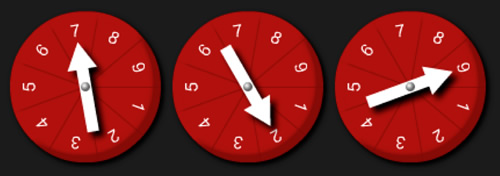#### You may also like### Pebbles

Place four pebbles on the sand in the form of a square. Keep adding as few pebbles as necessary to double the area. How many extra pebbles are added each time?### Bracelets

Investigate the different shaped bracelets you could make from 18 different spherical beads. How do they compare if you use 24 beads?### Sweets in a Box

How many different shaped boxes can you design for 36 sweets in one layer? Can you arrange the sweets so that no sweets of the same colour are next to each other in any direction?

# Division Rules

## Division Rules

This challenge is about dividing a three-digit number by a single-digit number.

Begin by deciding which number you are going to be dividing by. This is your divisor.
Your challenge is going to be to come up with some rules for this divisor.

Now generate a three-digit number. This is your dividend.

You could use the spinners here to generate the digits, you could use dice or could just use your imagination!Create other dividends and divide them by the same divisor. Record the answers.

Look carefully at the answers. When is the answer a whole number? When is there a remainder of 1?
Can you spot any patterns?
Can you come up with any rules?

#### Why do this activity?

This activity provides a context for practising dividing a three-digit number by a single-digit number. It encourages children to notice patterns and conjecture, and test rules. This may lead to establishing ways of recognising which multiplication tables three-digit numbers appear in.

#### Possible approach

The lesson may begin by recapping any patterns that children have recognised in the multiplication tables up to x10 or x12. They may know about numbers in the table of 5 always ending in a 5 or a 0 for example. This activity is about extending these rules to thinking about three-digit numbers.

Before the starting the task, it may be worth reminding children of the methods they could use for division.

As a class you could explore a divisor of 2 and record results as below:

 Calculation Whole number answer Remainder of 1 269 Ã· 2 = 134 r1 269 547 Ã· 2 = 273 r1 547 466 Ã· 2 = 233 466 728 Ã· 2 = 364 728

Encourage the class to look closely at the numbers resulting in different types of answer, to look for similarities and use this information to generate a rule for the types of numbers that appear in each column. Make a conjecture and then test it!

Remember that whole number answers mean that the dividend is a multiple of the divisor.

The class could then all explore the same divisor or different groups could look at different divisors (an opportunity to differentiate the activity).

Some children may move onto the extension task (below).

#### Key questions

What is the same about the numbers with a whole number answer?
Can you come up with a rule?
Do you think you could spot multiples of ...  without needing to complete the division calculation?

#### Possible extensions

Another way of approaching the problem is to keep the dividend constant and vary the divisors. So you could explore what happens when you divide 123 (for example) by 2, then by 3, then by 4 and so on. Can you find any three-digit prime numbers? Is there a way of spotting these numbers quickly?

#### Possible support

A multiplication grid and a calculator would be useful (the latter only if the emphasis is not on practising a written method).